# Navy STG Sonar Technician - Surface ASVAB Study Guide

 Branch Navy MOS STG Title Sonar Technician - Surface Description Operate (manipulate, control, evaluate, and interpret data) surface sonar and other oceanographic systems; operate surface ship underwater fire control systems (with associated equipment) for the solution of antisubmarine warfare problems; perform organizational and intermediate maintenance on surface sonar and allied equipment.Operate (manipulate, control, evaluate, and interpret data) surface sonar and other oceanographic systems.E4-E6; Able to perform the duties required for E1-E3;E7-E9; Able to perform the duties required for E4-E6; Supervises training of personnel in operation, maintenance, and repair of equipment; administers a long-range maintenance program; prepares quarterly maintenance schedules; plan, direct, organize, schedule. Subtests Arithmetic Reasoning, Electronics Information, General Science, Math Knowledge

# Arithmetic Reasoning

• 13 Questions
• 54 Problems
• 36 Flash Cards

### Fundamentals

##### Number Properties
###### Integers

An integer is any whole number, including zero. An integer can be either positive or negative. Examples include -77, -1, 0, 55, 119.

###### Rational Numbers

A rational number (or fraction) is represented as a ratio between two integers, a and b, and has the form $${a \over b}$$ where a is the numerator and b is the denominator. An improper fraction ($${5 \over 3}$$) has a numerator with a greater absolute value than the denominator and can be converted into a mixed number ($$1 {2 \over 3}$$) which has a whole number part and a fractional part.

###### Absolute Value

The absolute value is the positive magnitude of a particular number or variable and is indicated by two vertical lines: $$\left|-5\right| = 5$$. In the case of a variable absolute value ($$\left|a\right| = 5$$) the value of a can be either positive or negative (a = -5 or a = 5).

###### Factors & Multiples

A factor is a positive integer that divides evenly into a given number. The factors of 8 are 1, 2, 4, and 8. A multiple is a number that is the product of that number and an integer. The multiples of 8 are 0, 8, 16, 24, ...

###### Greatest Common Factor

The greatest common factor (GCF) is the greatest factor that divides two integers.

###### Least Common Multiple

The least common multiple (LCM) is the smallest positive integer that is a multiple of two or more integers.

###### Prime Number

A prime number is an integer greater than 1 that has no factors other than 1 and itself. Examples of prime numbers include 2, 3, 5, 7, and 11.

##### Operations on Fractions
###### Simplifying Fractions

Fractions are generally presented with the numerator and denominator as small as is possible meaning there is no number, except one, that can be divided evenly into both the numerator and the denominator. To reduce a fraction to lowest terms, divide the numerator and denominator by their greatest common factor (GCF).

Fractions must share a common denominator in order to be added or subtracted. The common denominator is the least common multiple of all the denominators.

###### Multiplying & Dividing Fractions

To multiply fractions, multiply the numerators together and then multiply the denominators together. To divide fractions, invert the second fraction (get the reciprocal) and multiply it by the first.

##### Operations on Exponents
###### Defining Exponents

An exponent (cbe) consists of coefficient (c) and a base (b) raised to a power (e). The exponent indicates the number of times that the base is multiplied by itself. A base with an exponent of 1 equals the base (b1 = b) and a base with an exponent of 0 equals 1 ( (b0 = 1).

To add or subtract terms with exponents, both the base and the exponent must be the same. If the base and the exponent are the same, add or subtract the coefficients and retain the base and exponent. For example, 3x2 + 2x2 = 5x2 and 3x2 - 2x2 = x2 but x2 + x4 and x4 - x2 cannot be combined.

###### Multiplying & Dividing Exponents

To multiply terms with the same base, multiply the coefficients and add the exponents. To divide terms with the same base, divide the coefficients and subtract the exponents. For example, 3x2 x 2x2 = 6x4 and $${8x^5 \over 4x^2}$$ = 2x(5-2) = 2x3.

###### Exponent to a Power

To raise a term with an exponent to another exponent, retain the base and multiply the exponents: (x2)3 = x(2x3) = x6

###### Negative Exponent

A negative exponent indicates the number of times that the base is divided by itself. To convert a negative exponent to a positive exponent, calculate the positive exponent then take the reciprocal: $$b^{-e} = { 1 \over b^e }$$. For example, $$3^{-2} = {1 \over 3^2} = {1 \over 9}$$

Radicals (or roots) are the opposite operation of applying exponents. With exponents, you're multiplying a base by itself some number of times while with roots you're dividing the base by itself some number of times. A radical term looks like $$\sqrt[d]{r}$$ and consists of a radicand (r) and a degree (d). The degree is the number of times the radicand is divided by itself. If no degree is specified, the degree defaults to 2 (a square root).

The radicand of a simplified radical has no perfect square factors. A perfect square is the product of a number multiplied by itself (squared). To simplify a radical, factor out the perfect squares by recognizing that $$\sqrt{a^2} = a$$. For example, $$\sqrt{64} = \sqrt{16 \times 4} = \sqrt{4^2 \times 2^2} = 4 \times 2 = 8$$.

To add or subtract radicals, the degree and radicand must be the same. For example, $$2\sqrt{3} + 3\sqrt{3} = 5\sqrt{3}$$ but $$2\sqrt{2} + 2\sqrt{3}$$ cannot be added because they have different radicands.

To multiply or divide radicals, multiply or divide the coefficients and radicands separately: $$x\sqrt{a} \times y\sqrt{b} = xy\sqrt{ab}$$ and $${x\sqrt{a} \over y\sqrt{b}} = {x \over y}\sqrt{a \over b}$$

###### Square Root of a Fraction

To take the square root of a fraction, break the fraction into two separate roots then calculate the square root of the numerator and denominator separately. For example, $$\sqrt{9 \over 16}$$ = $${\sqrt{9}} \over {\sqrt{16}}$$ = $${3 \over 4}$$

##### Miscellaneous
###### Scientific Notation

Scientific notation is a method of writing very small or very large numbers. The first part will be a number between one and ten (typically a decimal) and the second part will be a power of 10. For example, 98,760 in scientific notation is 9.876 x 104 with the 4 indicating the number of places the decimal point was moved to the left. A power of 10 with a negative exponent indicates that the decimal point was moved to the right. For example, 0.0123 in scientific notation is 1.23 x 10-2.

###### Factorials

A factorial has the form n! and is the product of the integer (n) and all the positive integers below it. For example, 5! = 5 x 4 x 3 x 2 x 1 = 120.

### Applications

##### Order of Operations
###### PEMDAS

Arithmetic operations must be performed in the following specific order:

1. Parentheses
2. Exponents
3. Multiplication and Division (from L to R)
4. Addition and Subtraction (from L to R)

The acronym PEMDAS can help remind you of the order.

###### Distributive Property - Multiplication

The distributive property for multiplication helps in solving expressions like a(b + c). It specifies that the result of multiplying one number by the sum or difference of two numbers can be obtained by multiplying each number individually and then totaling the results: a(b + c) = ab + ac. For example, 4(10-5) = (4 x 10) - (4 x 5) = 40 - 20 = 20.

###### Distributive Property - Division

The distributive property for division helps in solving expressions like $${b + c \over a}$$. It specifies that the result of dividing a fraction with multiple terms in the numerator and one term in the denominator can be obtained by dividing each term individually and then totaling the results: $${b + c \over a} = {b \over a} + {c \over a}$$. For example, $${a^3 + 6a^2 \over a^2} = {a^3 \over a^2} + {6a^2 \over a^2} = a + 6$$.

###### Commutative Property

The commutative property states that, when adding or multiplying numbers, the order in which they're added or multiplied does not matter. For example, 3 + 4 and 4 + 3 give the same result, as do 3 x 4 and 4 x 3.

##### Ratios
###### Ratios

Ratios relate one quantity to another and are presented using a colon or as a fraction. For example, 2:3 or $${2 \over 3}$$ would be the ratio of red to green marbles if a jar contained two red marbles for every three green marbles.

###### Proportions

A proportion is a statement that two ratios are equal: a:b = c:d, $${a \over b} = {c \over d}$$. To solve proportions with a variable term, cross-multiply: $${a \over 8} = {3 \over 6}$$, 6a = 24, a = 4.

###### Rates

A rate is a ratio that compares two related quantities. Common rates are speed = $${distance \over time}$$, flow = $${amount \over time}$$, and defect = $${errors \over units}$$.

###### Percentages

Percentages are ratios of an amount compared to 100. The percent change of an old to new value is equal to 100% x $${ new - old \over old }$$.

##### Statistics
###### Averages

The average (or mean) of a group of terms is the sum of the terms divided by the number of terms. Average = $${a_1 + a_2 + ... + a_n \over n}$$

###### Sequence

A sequence is a group of ordered numbers. An arithmetic sequence is a sequence in which each successive number is equal to the number before it plus some constant number.

###### Probability

Probability is the numerical likelihood that a specific outcome will occur. Probability = $${ \text{outcomes of interest} \over \text{possible outcomes}}$$. To find the probability that two events will occur, find the probability of each and multiply them together.

##### Word Problems
###### Practice

Many of the arithmetic reasoning problems on the ASVAB will be in the form of word problems that will test not only the concepts in this study guide but those in Math Knowledge as well. Practice these word problems to get comfortable with translating the text into math equations and then solving those equations.

# Electronics Information

• 104 Questions
• 9 Problems
• 77 Flash Cards

### Electricity

##### Electron Flow
###### Electrons

All electricity is the movement of electrons which are subatomic particles that orbit the nucleus of an atom. Electrons occupy various energy levels called shells and how well an element enables the flow of electrons depends on how many electrons occupy its outer (valence) electron shell.

###### Conductors

Conductors are elements that allow electrons to flow freely. Their valence shell is less than half full of electrons that are able to move easily from one atom to another.

###### Insulators

Insulators have valence shells that are more than half full of electrons and, as such, are tightly bound to the nucleus and difficult to move from one atom to another.

###### Semiconductors

Semiconductors have valence shells that are exacly half full and can conduct electricity under some conditions but not others. This property makes them useful for the control of electrical current.

###### Current

Current is the rate of flow of electrons per unit time and is measured in amperes (A). A coulomb (C) is the quantity of electricity conveyed in one second by a current of one ampere.

###### Voltage

Voltage (V) is the electrical potential difference between two points. Electrons will flow as current from areas of high potential (concentration of electrons) to areas of low potential. Voltage and current are directly proportional in that the higher the voltage applied to a conductor the higher the current that will result.

###### Resistance

Resistance is opposition to the flow of current and is measured in ohms (Ω). One ohm is defined as the amount of resistance that will allow one ampere of current to flow if one volt of voltage is applied. As resistance increases, current decreases as resistance and current are inversely proportional.

###### Conductive Materials

All conductors have resistance and the amount of resistance varies with the element. But, resistance isn't the only consideration when choosing a conductor as the most highly conductive elements like silver and gold are also more expensive and more brittle than slightly less conductive elements like copper. A balance needs to be struck between the electrical qualities of a material and its cost and durability.

###### Power

Electrical power is measured in watts (W) and is calculated by multiplying the voltage (V) applied to a circuit by the resulting current (I) that flows in the circuit: P = IV. In addition to measuring production capacity, power also measures the rate of energy consumption and many loads are rated for their consumption capacity. For example, a 60W lightbulb utilizes 60W of energy to produce the equivalent of 60W of heat and light energy.

##### Circuits

A load is a source of resistance that converts electrical energy into another form of energy. The components of a microwave, for example, are loads that work together to convert household electricity into radation that can be used to quickly cook food.

###### Open & Closed Circuits

A closed circuit is a complete loop or path that electricity follows. It consists of a source of voltage, a load, and connective conductors. If the circuit is interrupted, if a wire is disconnected or cut for example, it becomes an open circuit and no electricity will flow.

###### Ohm's Law

Ohm's law specifies the relationship between voltage (V), current (I), and resistance (R) in an electrical circuit: V = IR.

###### Series Circuit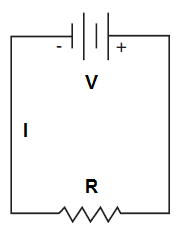A series circuit has only one path for current to flow. In a series circuit, current (I) is the same throughout the circuit and is equal to the total voltage (V) applied to the circuit divided by the total resistance (R) of the loads in the circuit. The sum of the voltage drops across each resistor in the circuit will equal the total voltage applied to the circuit.

###### Parallel Circuit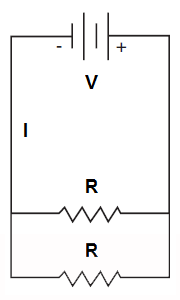In a parallel circuit, each load occupies a separate parallel path in the circuit and the input voltage is fully applied to each path. Unlike a series circuit where current (I) is the same at all points in the circuit, in a parallel circuit, voltage (V) is the same across each parallel branch of the circuit but current differs in each branch depending on the load (resistance) present.

###### Series-Parallel Circuits

Circuits are not limited to only series or only parallel configurations. Most circuits contain a mix of series and parallel segments. A good example is a household circuit breaker. Electrical outlets in each section of the house are wired in parallel with the circuit breaker for that section wired in series making it easy to cut off electricity to the parallel parts of the circuit when needed.

###### Battery Configurations

Batteries can be connected together in various combinations to increase their total voltage and/or total current. Connecting batteries in series combines their voltage while keeping their current the same, connecting batteries in parallel combines their current while keeping their voltage the same, and using a series-parallel configuration, half the batteries can be connected in series and half in parallel to combine both voltage and current.

### Electrical Systems

##### Types of Current
###### Direct Current (DC)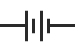Direct current flows in only one direction in a circuit, from the negative terminal of the voltage source to the positive. A common source of direct current (DC) is a battery.

###### Alternating Current (AC)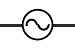In contrast to the constant one-way flow of direct current, alternating current changes direction many times each second. Electricity is delivered from power stations to customers as AC because it provides a more efficient way to transport electricity over long distances.

##### Electronic Components
###### Resistors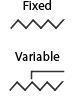Resistors are used to limit voltage and/or current in a circuit and can have a fixed or variable resistance. Variable resistors (often called potentiometers or rheostats) are used when dynamic control over the voltage/current in a circuit is needed, for example, in a light dimmer or volume control.

###### Fuses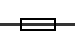Fuses are thin wires that melt when the current in a circuit exceeds a preset amount. They help prevent short circuits from damaging circuit components when an unusually large current is applied to the circuit, either through component failure or spikes in applied voltage.

###### Circuit Breakers

Like fuses, circuit breakers stop current flow once it reaches a certain amount. They have the advantage of being reusable (fuses must be replaced when "blown") but respond more slowly to current surges and are more expensive than fuses.

###### Capacitors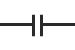Capacitors store electricity and are used in circuits as temporary batteries. Capacitors are charged by DC current (AC current passes through a capacitor) and that stored charge can later be dissipated into the circuit as needed. Capacitors are often used to maintain power within a system when it is disconnected from its primary power source or to smooth out or filter voltage within a circuit.

###### Diodes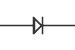A diode allows current to pass easily in one direction and blocks current in the other direction. Diodes are commonly used for rectification which is the conversion of alternating current (AC) into direct current (DC). Because a diode only allows current flow in one direction, it will pass either the upper or lower half of AC waves (half-wave rectification) creating pulsating DC. Multiple diodes can be connected together to utilize both halves of the AC signal in full-wave rectification.

###### TransistorsThe transistor is the foundation of modern electronic devices. It is made entirely from semiconductor material (making it a solid state device) and can serve many different functions in a circuit including acting as a switch, amplifier, or current regulator. A transistor works by allowing a small amount of current applied at the base to control general current flow from collector to emitter through the transistor.

###### Integrated Circuits

Circuits containing transistors are packaged into integrated circuit chips that allow encapsulating complex circuit designs (CPU, memory, I/O) for easier integration into electronic devices and machines.

###### Thermocouples

A thermocouple is a temperature sensor that consists of two wires made from different conductors. The junction of these two wires produces a voltage based on the temperature difference between them.

##### Magnetism
###### Magnetic Fields

A moving electric current produces a magnetic field proportional to the amount of current flow. This magnetic field can be made stronger by winding the wire into a coil and further enhanced if done around an iron containing (ferrous) core.

###### Inductors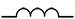An inductor is coiled wire that stores electric energy in the form of magnetic energy and resists changes in the electric current flowing through it. If current is increasing, the inductor produces a voltage that slows the increase and, if current is decreasing, the magnetic energy in the coil opposes the decrease to keep the current flowing longer. In contrast to capacitors, inductors allow DC to pass easily but resist the flow of AC.

###### Transformers

A transformer utilizes an inductor to increase or decrease the voltage in a circuit. AC flowing in a coil wrapped around an iron core magnetizes the core causing it to produce a magnetic field. This magnetic field generates a voltage in a nearby coil of wire and, depending on the number of turns in the wire of the primary (source) and secondary coils and their proximity, voltage is induced in the secondary coil.

# General Science

• 264 Questions
• 315 Flash Cards

### Nutrition & Health

##### Macronutrients
###### Proteins

Found in both animal sources (meat, fish, eggs, cheese) and vegetables (beans, nuts, some grains), proteins are important for the body's maintenance, growth, and repair.

###### Carbohydrates

Carbohydrates are major sources of energy for the body and are found in sugars (fruit, cane sugar, beets) and starches (bread, rice, potatoes, pasta).

###### Fats

Like carbohydrates, fats provide energy to the body. The difference is energy from fats tends to be longer burning as opposed to the quick fuel provided by carbohydrates. Fats come in three types, saturated (meats, shellfish, eggs, milk), monounsaturated (olives, almonds, avocados), and polyunsaturated (vegetable oils). Saturated fats can raise LDL ("bad") cholesterol while unsaturated fats can decrease it.

##### Micronutrients
###### Minerals

Small quantities of certain minerals like iron, calcium, magnesium, and salt are important for nutrition and health.

###### Vitamins

Vitamins are necessary for a wide variety of bodily processes. Some vitamins like Vitamins A and C come from diet but others, like Vitamin D, are generated in response to sunlight.

###### Fiber

Fiber provides bulk to help the large intestine carry away waste. Good sources of fiber are leafy vegetables, beans, potatoes, fruits, and whole grains.

##### Nutritional Deficiencies

A diet lacking healthy amounts of necessary nutrients can lead to a variety of health conditions and diseases. Examples include anemia which is caused by a lack of iron and scurvy which is caused by a lack of Vitamin C.

###### Health Benefits of Vitamins & Minerals
Vitamin / Mineral Sources Health Benefits
Calcium Dairy products (milk, yogurt, cheese), spinach. Aids bone growth and repair, muscle function.
Iron Red meat, beans, whole grains. Allows red blood cells to transfer oxygen to body tissues.
Magnesium Nuts, whole grains, green leafy vegetables. Muscle, nerve, and enzyme function.
Potassium Bananas, nuts, seeds. Helps balance fluid levels in the body.
Vitamin A Liver, milk, eggs, carrots. Vision, immune system, cell growth.
Vitamin C Green and red peppers, citrus fruits, broccoli. Collagen formation, immune system function, antioxidant (helps protect cells from damage).
Vitamin D Exposure to sunlight. Helps calcium strengthen bones, muscle, nerve, and immune system function.

### Human Body Systems

##### Skeleton & Muscles
###### Exoskeleton

An exoskeleton (external skeleton) is common in arthropods like insects, spiders, and crustaceans.

###### Endoskeleton

An endoskeleton (internal skeleton) is a charateristic of vertebrate animals, including humans.

###### Bones & Cartilage

Hard bones provide primary support for the endoskeleton while more flexible cartilage is found at the end of all bones, at the joints, and in the nose and ears. In addition to providing support and protecting bodily organs, bones also produce blood cells and store minerals like calcium.

###### Tendons & Ligaments

Tough fibrous cords of connective tissue called tendons connect muscles to the skeleton while another type of connective tissue called ligaments connect bones to other bones at joints (elbow, knee, fingers, spinal column).

##### Respiratory System
###### Respiration

The respiratory system manages respiration which is the process by which blood cells absorb oxygen and eliminate carbon dioxide.

###### Filtering Air

After air enters through the nose, it passes through the nasal cavity which filters, moistens, and warms it. Further filtering takes place in the pharynx, which also helps protect against infection, and then in the trachea which is just past the epiglottis, responsible for preventing food from entering the airway.

###### Lungs

The trachea branches into the left and right bronchi which each lead to a lung where the bronchi subdivide into smaller tubes called bronchioles. Each bronchiole ends in a small sac called an alveolus which allows oxygen from the air to enter the bloodstream via tiny blood vessels called capillaries.

###### Breathing

The diaphragm is a system of muscles that allows breathing. During inhalation, the diaphragm expands and air rushes in to fill the space created. Then, during exhalation, the diaphragm contracts and forces the air back out.

##### Circulatory System
###### Circulation

Like the respiratory system, the circulatory system serves to transport oxygen throughout the body while removing carbon dioxide. In addition, the circulatory system transports nutrients from the digestive system.

###### Heart

The heart is the organ that drives the circulatory system. In humans, it consists of four chambers with two that collect blood called atria and two that pump blood called ventricles. The heart's valves prevent blood pumped out of the ventricles from flowing back into the heart.

###### Pulmonary Artery & Vein

The two largest veins in the body, the venae cavae, pass blood to the right ventricle which pumps the blood to the lungs through the pulmonary artery. Blood picks up oxygen in the lungs and returns it to the left atrium via the pulmonary vein.

###### Blood Flow

To provide oxygen to the body, blood flows through the heart in a path formed by the right atrium → right ventricle → lungs → left atrium → left ventricle → body. When blood enters the right side of the heart it is deoxygenated. It enters the left side of the heart oxygenated after traveling to the lungs.

###### Arteries

The aorta is the body's largest artery and receives blood from the pulmonary vein via the left ventricle. From there, blood is circulated through the rest of the body through smaller arteries called arterioles that branch out from the heart. Finally, blood is delivered to bodily tissues through capillaries.

###### Veins

Veins carry blood back to the heart from the body. While arteries are thick-walled because they carry oxygenated blood at high pressure, veins are comparatively thin-walled as they carry low-pressure deoxygenated blood. Like the heart, veins contain valves to prevent blood backflow.

###### Capillaries

Capillaries are small thin-walled vessels that permit the exchange of oxygen, carbon dioxide, nutrients, and waste between blood and the body's cells. This process of exchange is called diffusion.

###### Blood Cells

Blood is created in bone marrow and is made up of cells suspended in liquid plasma. Red blood cells carry oxygen, white blood cells fight infection, and platelets are cell fragments that allow blood to clot.

###### Blood Types

Blood is categorized into four different types (A, B, AB, and O) based on the type of antigens found on the outside of the red blood cells. Additionally, each type can be negative or positive based on whether or not the cells have an antigen called the Rh factor.

###### Blood Transfer

Blood transfer is limited by the type and Rh factor of the blood. Someone who has Rh-factor negative blood cannot receive blood with a positive type but a person with Rh-factor positive type blood can receive Rh-negative blood. Type O negative blood is the universal donor because it can be given to a person with any blood type. Type AB positive is the universal recipient meaning someone with this blood type can receive any other type of blood.

##### Digestive System
###### Mouth & Throat

Digestion begins in the mouth where the teeth and tongue break down food mechanically through chewing and saliva, via the enzyme salivary amylase, starts to break starches down chemically. From the mouth, food travels down the esophagus where contractions push the food into the stomach.

###### Stomach

Food is mixed with gastric acid and pepsin in the stomach to help break down protein.

###### Small Intestine

The small intestine is where most digestion takes place. As food travels along the small intestine it gets broken down completely by enzymes secreted from the walls. These enzymes are produced in the small intestine as well as in the pancreas and liver. After the enzymes break down the food, the resulting substances are then absorbed into the blood via capillaries in the small intestine walls.

###### Pancreas

The acids produced by the pancreas contain several enzymes that aid in digestion. Lipase converts fat to glycerol and fatty acids. Pancreatic amylase breaks down complex carbohydrates into simple sugars. Trypsin converts polypeptides (the building blocks of protein) into amino acids.

###### Liver

The liver produces bile which emulsifies (separates) fat.

###### Large Intestine

The large intestine (colon) follows the small intestine and processes the physical waste produced by digestion, absorbing water and minerials that remain back into the body. Solid waste is then stored in the rectum while liquid waste is stored in the bladder.

Chemical waste like excess water, minerals, and salt are filtered from the blood by the kidneys and secreted into the urine. Urine is transported from the kidneys to the bladder through ureters.

##### Nervous System
###### Central & Peripheral Nervous Systems

The nervous system consists of the brain and spinal cord (central nervous system) and the peripheral nervous system which is the network of nerve cells (neurons) that collect and distribute signals from the central nervous system throughout the body.

###### Cerebrum

The cerebrum is the major part of the brain and is responsible for the main senses (thinking, hearing, seeing).

###### Cerebellum

The cerebellum is a large cluster of nerves at the base of the brain that's responsible for balance, movement, and muscle coordination.

###### Medulla

Part of the brainstem, the medulla is the connection between the brain and the spinal cord. It controls involuntary actions like breathing, swallowing, and heartbeat.

###### Spinal Cord

The spinal cord connects the brain to the body's network of nerves. It carries impulses between all organs and the brain and controls simple reflexes.

###### Somatic Nervous System

Part of the peripheral nervous system, the somatic nervous system is made up of nerve fibers that send sensory information to the central nervous system and control voluntary actions.

###### Autonomic Nervous System

Part of the peripheral nervous system, the autonomic nervous system regulates involuntary activity in the heart, stomach, and intestines.

##### Reproductive System
###### Ovulation

Approximately every 28 days during female ovulation an egg (ovum) is released from one of the ovaries and travels through the oviduct (fallopian tube) and into the uterus. At the same time, the endometrial lining of the uterus becomes prepared for implantation.

###### Reproduction

During intercourse, the penis ejaculates sperm, produced in the testes, into the vagina. Some of the sperm makes their way to the uterus where, if they encounter an egg to fertilize, unite with the ovum to form a fertilized egg or zygote. The zygote then may implant in the uterus and eventually develop into a fetus.

###### Menstruation

If the ovum fails to become fertilized, the lining of the uterus sloughs off during menstruation. From puberty to menopause, this cycle of menstruation repeats monthly (except during pregnancy).

### Cellular Biology

##### Genetics
###### Heredity

Heredity is the passing on of physical or mental characteristics genetically from one generation to another. Heredity is made possible via large strings of chromosomes which carry information encoded in genes.

###### Meiosis

Reproductive (haploid) cells known as gametes have half as many (23) pairs of chromosomes as normal (diploid) cells. When the male gamete (sperm) combines with the female gamete (ovum) through meiosis to form a zygote, each gamete supplies half the chromosomes needed to form the normal diploid cells.

###### DNA

Deoxyribonucleic acid (DNA) is the molecule that contains genetic information. DNA is encoded through a combination of nucleotides that bind together in a specific double helix pattern.

###### Genes

The gene is the base unit of inheritance and is contained within DNA. A gene may come in several varieties (alleles) and there are a pair of alleles for every gene. If the alleles are alike, a person is homozygous for that gene. If the alleles are different, heterozygous.

###### Traits

The traits represented by genes are inherited independently of each other (one from the male and one from the female gamete) and a trait can be dominant or recessive. A dominant trait will be expressed when paired with a recessive trait while two copies of a recessive trait (one from each parent) must be present for the recessive trait to be expressed.

###### Genetic Type

A person's genotype is their genetic makeup and includes both dominant and recessive alleles. Phenotype is how the genes express themselves in physical characteristics.

##### Cell Structures
###### Nucleus

Cells are classified into one of two groups based on whether or not they have a nucleus. Eukaryotic cells have a nucleus, prokaryotic cells do not have a nucleus and therefore have a less complex structure than eukaryotic cells.

###### Cytoplasm

The nucleus of a eukaryotic cell contains the genetic material of the cell and is surrounded by cytoplasm which contains many organelles. These include:

Organelle Function
ribosomes produce proteins
mitochondria produce energy
endoplasmic reticulum helps synthesize proteins and fats
Golgi apparatus prepare proteins for use
lysosomes help the cell manage waste
centrosomes guide cell reproduction

###### Cell Membrane

Animal cells are surrounded by a semipermeable membrane which allows for the transfer of water and oxygen to and from the cell. In plant cells, the cell membrane is surrounded by a somewhat rigid cell wall which provides the cell structure and support.

###### Cell Energy

Some plant cells produce their own energy through photosynthesis which is the process by which sunlight, carbon dioxide, and water react to make sugar and oxygen. Animal cells cannot produce their own energy and, instead, generate energy when mitochondria consume outside sugar and oxygen through aerobic respiration.

###### Fermentation

If no oxygen is present, cellular respiration is anaerobic and will result in fermentation where either lactic acid or alcohol is used instead of oxygen.

###### Cell Division

Cell division is the process by which cells replicate genetic material in the nucleus. Cell division consists of several phases:

Phase Major Process
interphase chromosomes replicate into chromatids and the cell grows
prophase chromatids pair up
metaphase paired chromatids move to opposite sides of the cell
anaphase cell elongates and nucleus begins to separate
telophase separation of nucleus is complete resulting in two new nuclei
cytokinesis cytoplasm and cell membranes complete their separation resulting in two separate cells

### Ecology

##### Classifications
###### Biosphere

The biosphere is the global ecological system integrating all living beings and their relationships. This includes their interactions with the lithosphere (the rigid outer part of the earth, consisting of the crust and upper mantle), hydrosphere (all surface water), and atmosphere (the envelope of gases surrounding the planet).

###### Biome

A biome is a large naturally occurring community of flora (plants) and fauna (animals) occupying a major habitat (home or environment).

###### Ecosystem

An ecosystem is a biological community of interacting organisms and their physical environment. This includes both the biotic (living) and abiotic (non-living).

###### Species Groups

A population is a group of organisms of the same species who live in the same area at the same time. A community is a group of populations living and interacting with each other in an area.

##### Food Chain
###### Producers

Producers (autotrophs) serve as a food source for other organisms. Typical producers are plants that can make their own food through photosynthesis and certain bacteria that are capable of converting inorganic substances into food through chemosynthesis

###### Decomposers

Decomposers (saprotrophs) are organisms such as bacteria and fungi that break down the organic matter in the dead bodies of plants and animals into simple nutrients.

###### Scavengers

Like decomposers, scavengers also break down the dead bodies of plants and animals into simple nutrients. The difference is that scavengers operate on much larger refuse and dead animals (carrion). Decomposers then consume the much smaller particles left over by the scavengers.

###### Consumers

Most animals consume other organisms to survive. Consumers (heterotrophs) are divided into three types, primary, secondary, and tertiary, based on their place in the food chain.

###### Primary Consumers

Primary consumers (herbivores) subsist on producers like plants and fungus. Examples are grasshoppers, cows, and plankton.

###### Secondary Consumers

Secondary consumers (carnivores) subsist mainly on primary consumers. Omnivores are secondary consumers that also eat producers. Examples are rats, fish, and chickens.

###### Tertiary Consumers

Tertiary consumers eat primary consumers and secondary consumers and are typically carnivorous predators. Tertiary consumers may also be omnivores. Examples include wolves, sharks, and human beings.

##### Taxonomy
###### Domain

The broadest classification of life splits all organisms into three groups called domains. The three domains of life are bacteria, archaea and eukaryota.

###### Kingdom

Below domain, life is classified into six kingdoms: plants, animals, archaebacteria, eubacteria, and fungi. The last kingdom, protists, include all microscopic organisms that are not bacteria, animals, plants or fungi. (Archaebacteria and eubacteria are sometimes combined into a single kingdom, monera.)

###### Classification of Life

Classifications of life are too numerous to enumerate, here's an overview of the classifications from broadest to narrowist:

Classification Contains Related
Kingdom phyla
Phylum classes
Class orders
Order families
Family genera
Genus species
Species organisms

###### Species

The narrowest classification of life, species, contains organisms that are so similar that they can only reproduce with others of the same species.

### Earth Science

##### Structure of the Earth
###### Crust

The crust is the Earth's outermost layer and is divided into oceanic and continental types. Oceanic crust is 3 miles (5 km) to 6 miles (10 km) thick and is composed primarily of denser rock. Continental crust is 20 to 30 miles (30 to 50 km) thick and composed primarily of less dense rock. The crust makes up approximately one percent of the Earth's total volume.

###### Mantle

Mantle makes up 84% of the Earth's volume and has an average thickness of approximately 1,800 miles (2,900 km). It is dense, hot, and primarily solid although in places it behaves more like a viscous fluid as the plates of the upper mantle and crust gradually "float" along its circumference.

###### Core

The Earth's core is divided into the liquid outer core (1,430 miles or 2,300 km radius) and the solid inner core (745 miles or 1,200 km radius).

###### Plate Tectonics

The crust and the rigid lithosphere (upper mantle) is made up of approximately thirty separate plates. These plates more very slowly on the slightly more liquid mantle (asthenosphere) beneath them. This movement has resulted in continental drift which is the gradual movement of land masses across Earth's surface. Continental drift is a very slow process, occurring over hundreds of millions of years.

###### Types of Rock

The Earth's rocks fall into three categories based on how they're formed. Igneous rock (granite, basalt, obsidian) is formed from the hardening of molten rock (lava), sedimentary rock (shale, sandstone, coal) is formed by the gradual despositing and cementing of rock and other debris, and metamorphic rock (marble, slate, quartzite) which is formed when existing rock is altered though pressure, temperature, or chemical processes.

###### Geologic Time Scale

The Earth is approximately 4.6 billion years old and its history is divided into time periods based on the events that took place and the forms of life that were dominant during those periods. The largest graduation of time is the eon and each eon is subdivided into eras, eras into periods, periods into epochs, and epochs into ages.

###### Cambrian Period

The Cambrian period is one of the most significant geological time periods. Lasting about 53 million years, it marked a dramatic burst of changes in life on Earth known as the Cambrian Explosion. It is from this period that the majority of the history of life on Earth, as documented by fossils, is found. Called the fossil record, the layering of these mineralized imprints of organisms preserved in sedementary rock have allowed geologists to build a historical record of plant and animal life on Earth.

##### Biochemical Cycles
###### Water Cycle

The water (hydrologic) cycle describes the movement of water from Earth through the atmosphere and back to Earth. The cycle starts when water evaporates into a gas from bodies of water like rivers, lakes and oceans or transpirates from the leaves of plants.

###### Precipitation

Rising into the atmosphere, the water condenses into clouds. When the clouds become too saturated with water, the water is released as snow or ice precipitation which may warm as it falls to reach Earth as rain.

###### Infiltration

The water then accumulates as runoff and eventually returns to bodies of water or is absorbed into the Earth (infiltration) and becomes part of the water table, an underground resevoir of fresh water.

###### Carbon Cycle

The carbon cycle represents the ciruit of carbon through Earth's ecosystem. Carbon dioxide (CO2) in the atmosphere is absorbed by plants through photosynthesis. Plants then die and release carbon back into the atmosphere during decomposition or are eaten by animals who breathe (respiration) the carbon into the atmosphere they exhale and produce waste which also releases carbon as it decays.

##### Atmosphere
###### Troposphere

The Earth's atmosphere has several layers starting with the troposphere which is closest in proximity to the surface. Containing most of the Earth's breathable air (oxygen and nitrogen), it's a region with warmer temperatures closer to the surface and cooler temperatures farther away which results in the rising and falling air that generates weather.

###### Stratosphere

The stratosphere is just above the troposphere and is stratified in temperature with warmer layers higher and cooler layers closer to Earth. This increase in temperature is a result of absorption of the Sun's radiation by the ozone layer.

###### Mesosphere

In the mesosphere, temperature again drops as altitude increases until the coldest point in the Earth's atmosphere, the mesopause, is reached where temperatures fall to −225 °F (−143 °C).

###### Thermosphere

Temperatures again increase with altitude in the thermosphere which is the hottest (4,530 °F / 2,500 °C) atmospheric layer due to direct exposure to the Sun's radiation. However, the gas in this layer is highly diluted so even though the atoms of gas may be very high in temperature, there are too few of them to effectively transfer much heat.

##### Weather
###### Fronts

An air mass is a large body of air that has similar moisture (density) and temperature characteristics. A front is a transition zone between two air masses.

###### Cold Front

A cold front is a warm-cold air boundary with the colder air replacing the warmer. As a cold front moves into an area, the heavier cool air pushes under the lighter warm air that it is replacing. The warm air becomes cooler as it rises and, if the rising air is humid enough, the water vapor it contains will condense into clouds and precipitation may fall.

###### Warm Front

A warm front is the boundary between warm and cool (or cold) air when the warm air is replacing the cold air. Warm air at the surface pushes above the cool air mass creating clouds and storms.

###### Stationary Front

When two air masses meet and neither is displaced, a stationary front is created. Stationary fronts often cause persistent cloudy wet weather.

###### Stratus Clouds

Clouds are categorized based on their shape, size, and altitude. Stratus clouds are low-altitude clouds characterized by horizontal layering with a broad flat base. When stratus clouds occur on the ground the result is fog.

###### Cumulus Clouds

Cumulus clouds are large, puffy, mid-altitude clouds with a flat base and a rounded top. These clouds grow upward and can develop into a cumulonimbus or thunderstorm cloud.

###### Cirrus Clouds

Cirrus clouds are thin, wispy high-altitude clouds composed of ice crystals that originate from the freezing of supercooled water droplets. Cirrus clouds generally occur in fair weather and point in the direction of air movement at their elevation.

##### Solar System
###### The Sun

The Sun is a G-type main-sequence star (G2V) but is informally known as a yellow dwarf star. Composed of 73% hydrogen and 25% helium, the hot plasma that makes up the Sun reaches 9,900°F (5,505°C) at the surface. It formed approximately 4.6 billion years ago and makes up 99.86% of the mass in the solar system.

###### Terrestrial Planets

The four planets closest to the Sun (Mercury, Venus, Earth, and Mars) are called terrestrial (Earth-like) planets because, like the Earth, they're solid with inner metal cores covered by rocky surfaces.

###### Outer Planets

In contrast to the solid terrestrial planets, the outer planets (Jupiter, Saturn, Uranus, and Neptune) consist of hydrogen and helium gas and water.

###### Asteroids

The solar system also contains over a million rocky fragments of at least 1km in diameter called asteroids as well as millions more with smaller diameters. Many of these asteroids are an asteroid belt between the orbits of Mars and Jupiter.

###### Kuiper Belt

The Kuiper Belt is similar to the asteroid belt but much larger. Extending beyond the orbit of Neptune, it contains objects composed mostly of frozen methane, ammonia, and water. Most notably, the Kuiper Belt is home to Pluto, a dwarf planet that, until a 2006 reclassification, was considered the ninth planet of the solar system.

###### Comets

A comet is a loose collection of ice, dust, and small rocky particles that, in contrast to an asteroid, has an extended atmosphere surrounding the center. When passing close to the Sun, this atmosphere warms and begins to release gases forming a visible coma or tail.

###### Meteoroids

Smaller rocks shed by asteroids and comets are called meteoroids. When these rocks reach Earth's atmosphere, they burn up in the mesosphere and become meteors. If a meteor manages to reach the Earth, it is called a meteorite.

###### Moon

Tides are caused by the gravitational interaction of Earth and the Moon.

### Physical Science

##### Metric System
###### Number System

The metric system is a number system that designates one base unit for each type of measurement. For example, the base unit for length is the meter and the base unit for mass is the gram.

###### Prefixes

A prefix is added to the base units of the metric system to indicate variations in size. Each prefix specifies a value relative to the base unit in a multiple of 10. Common prefixes are:

Prefix Symbol Relative Value Example
mega M 106 (1,000,000) Mm
kilo k 103 (1,000) km
base unit N/A 1 m
centi c 10-2 (1/100) cm
milli m 10-3 (1/1,000) mm
###### Base Units
Measurement Base Unit Example
length / distance meter (m) km
mass gram (g) kg
volume liter (L) mL
volume (medical) cubic centimeter (cc) cc
time second (s), minute (min), hour (h) ms, min, h

###### Fahrenheit Scale

More familiar in the United States is the Fahrenheit scale in which the freezing point of water is 32°F (0°C) and the boiling point is 212°F (100°C). To convert from C° to F° use the formula:

$$F° = {9 \over 5}C° + 32$$

and to convert from F° to C° use:

$$C° = {5 \over 9} (F° - 32)$$

###### Kelvin Scale

In contrast to the Celsius scale (measured in degrees centigrade) that fixes 0° at the freezing point of water and the Fahrenheit scale that uses 32°, the Kelvin scale fixes 0° at absolute zero (-273°C) which is the lowest temperature possible in the universe.

##### Motion
###### Velocity

Velocity is the rate at which an object changes position. Rate is measured in time and position is measured in displacement so the formula for velocity becomes $$\vec{v} = { \vec{d} \over t }$$

###### Vectors

Velocity and displacement are vector quantities which means each is fully described by both a magnitude and a direction. In contrast, scalar quantities are quantities that are fully described by a magnitude only. A variable indicating a vector quantity will often be shown with an arrow symbol: $$\vec{v}$$

###### Momentum

Momentum is a measure of how difficult it is for a moving object to stop and is calculated by multiplying the object's mass by its velocity: $$\vec{p} = m\vec{v}$$. Like velocity, momentum is a vector quantity as it expresses force applied in a specific direction.

###### Acceleration

Acceleration is the rate of change of velocity per unit of time. In physics, the delta symbol ($$\Delta$$) represents change so the formula for acceleration becomes $$\vec{a} = { \Delta \vec{v} \over t }$$

##### Forces and Energy
###### Force

Force is applied to change an object's speed or direction of motion.

###### Weight

Weight is a force that describes the attraction of gravity on an object. Force is measured in newtons (N) with 1 N being the force required to impart an acceleration of 1 m/s2 to a mass of 1 kg.

###### Mass vs. Weight

Mass is the amount of matter something has while weight is the force exerted on an object's mass by gravity. So, although a person's mass doesn't change when going from the Earth to the Moon, their weight will decrease because the force of the Moon's gravity is much less than that of Earth.

###### Work

Work is performed on an object when an applied force causes displacement along the same vector. Measured in joules (J) or newton-meters (Nm), work is calculated by multiplying force times displacement: $$W = \vec{F}\vec{d}$$

###### Power

Power is the rate at which work is performed or work per unit time: $$P = {w \over t}$$ and is measured in watts (W).

###### Kinetic Energy

Kinetic energy is the energy posessed by a moving object. Potential energy is stored energy in a stationary object based on its location, position, shape, or state.

###### Electromagnetic Spectrum

The electromagnetic spectrum covers all possible wavelengths and frequencies of radiation. From lowest frequency (longest wavelength) to highest frequency (shortest wavelength) radiation: radio waves → microwaves → infrared waves → visible light → ultraviolet light → X-rays → gamma rays.

###### Magnetism

Simple magnets have two poles, north and south, and opposite poles attract each other (N attracts S, S attracts N). Likewise, the same pole of two magnets repel (N repels N, S repels S). The Earth has a magnetic field and North and South Poles which enables the use of a magnetic compass to determine direction.

##### Newton's Laws
###### First Law of Motion

Also known as the law of inertia, Newton's first law of motion states that An object at rest stays at rest and an object in motion stays in motion with the same speed and in the same direction unless acted upon by an unbalanced force.

###### Second Law of Motion

Newton's second law of motion states that The acceleration of an object as produced by a net force is directly proportional to the magnitude of the net force, in the same direction as the net force, and inversely proportional to the mass of the object. This law basically means that the greater the mass of an object, the more force is needed to overcome its inertia.

###### Acceleration

Newton's second law of motion leads to the formula for acceleration which is a measure of the rate of change of velocity per unit time and, if you solve for positive acceleration, reveals how much net force is needed to overcome an object's mass. The formula for acceleration is $$\vec{a} = { \vec{F} \over m }$$ or, solving for force, $$\vec{F} = m\vec{a}$$.

###### Third Law of Motion

Newton's third law of motion states that For every action, there is an equal and opposite reaction. When an object exerts a force on another object, the second object exerts a force of equal magnitude in the opposite direction on the first object.

###### Law of Universal Gravitation

Newton's law of universal gravitation defines gravity: All objects in the universe attract each other with an equal force that varies directly as a product of their masses, and inversely as a square of their distance from each other. Expressed as a formula: $$\vec{F_{g}} = { Gm_{1}m_{2} \over r^2}$$ where r is the distance between the two objects and G is the gravitational constant with a value of 6.67 x 10-11.

##### Sound Waves
###### Vibration

A vibrating object produces a sound wave that travels outwardly from the object through a medium (any liquid or solid matter). The vibration disturbs the particles in the surrounding medium, those particles disturb the particules next to them, and so on, as the sound propagates away from the vibration.

###### Speed

The speed of a sound wave will vary with the medium. Sound travels fastest through media that has particles that are very close together, like metal. Thus, it travels faster through water than through air and doesn't travel at all through a vacuum (there are no particles in empty space to vibrate).

###### Frequency

The rate of vibration of sound is called frequency and is measured in hertz (Hz). One hertz is one repetition per second and sounds with high frequency have a higher pitch than sounds with lower frequency. Humans can hear sounds in the range of 20 Hz to 20 kHz.

###### Doppler Effect

The Doppler effect occurs when the source or listener (or both) of sound waves is moving. If they're moving closer together, the listener perceives the sound with a higher pitch and, when they're moving apart, the listener perceives the sound with a lower pitch.

##### Optics
###### Light Waves

Unlike mechanical sound waves that require a physical medium for propagation, light waves are electromagnetic and can travel through empty space. Light waves are also much faster, travelling at 186,000 m/s vs. 343 m/s for sound waves.

###### Refractive Index

The speed of light varies based on the material that the waves are passing through. The refractive index of a material indicates how easily light travels through it compared to how easily light travels through a vacuum. For example, the refractive index of water is 1.33, meaning that light travels 1.33 times faster in a vacuum than it does in water.

###### Refraction

Because different materials have different refractive indices, light changes speed when passing from one material to another. This causes the light to bend (refraction) at an angle that depends on the change in refractive index between the materials. The greater the difference, the higher the angle of refraction.

###### Reflection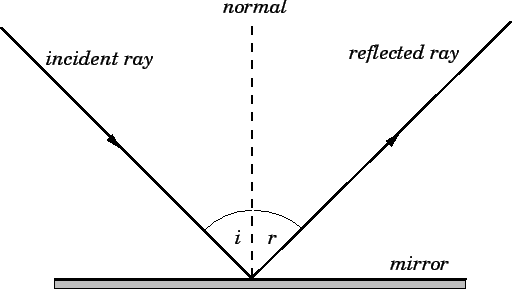The law of reflection specifies how waves, including light waves, bounce off of surfaces. Specifically, the angle of incidence of the approaching wave is equal to the angle of reflection of the reflected wave as measured from a line perpendicular (90°) to the surface.

###### Curved Mirrors

A concave (or converging) mirror bulges inward and focuses reflected light on the mirror's focal point where the mirror's angles of incidence converge. In contrast, a convex (or diverging) mirror bulges outward and diffuses the light waves that strike it. A common use of a concave mirror is in a reflecting telescope, a common use of a convex mirror is in the side view mirror of a car.

###### Curved Lenses

Unlike curved mirrors that operate on the principle of reflection, lenses utilize refraction. A convex lens is thicker in the middle than on the edges and converges light while a concave lens is thicker on the edges than in the middle and diffuses light. A common use for curved lenses is in eye glasses where a convex lens is used to correct farsightedness and a concave lens is used to correct nearsightedness.

##### Heat
###### Conduction

Heat is always transferred from warmer to cooler environments and conduction is the simplest way this transfer can occur. It is accomplished through direct contact between materials and materials like metals that transfer heat efficiently are called conductors while those that conduct heat poorly, such as plastic, are called insulators.

###### Convection

Convection is the transfer of heat by the circulation or movement of the heated parts of a liquid or gas. Examples of heat transfer by convection include water coming to a boil on a stove, ice melting, and steam from a cup of coffee.

Radiation occurs when electromagnetic waves transmit heat. An example is the heat from the Sun as it travels to Earth.

##### Matter
###### Element

An element is matter than cannot be separated into different types of matter by ordinary chemical methods.

###### Atom

An atom is the smallest component of an element that still retains the properties of the element.

###### Proton

A proton is a subatomic particle found in the nucleus of an atom. It carries a positive electric charge.

###### Neutron

A neutron is a subatomic particle found in the nucleus of an atom. It is neutral as it carries no electric charge.

###### Electron

An electron is a subatomic particle that orbits the nucleus of an atom. It carries a negative electric charge. Generally, an atom has the same number of negative electrons orbiting the nucleus as it does positive protons inside.

###### Compound

A compound is a substance containing two or more different chemical elements bound together by a chemical bond. In covalent compounds, electrons are shared between atoms. In ionic compounds, one atom borrows an electron from another atom resulting in two ions (electrically charged atoms) of opposite polarities that then become bonded electrostatically.

###### Molecule

A molecule is the smallest multi-atom particle of an element or compound that can exist and still retain the characteristics of the element or compound. The molecules of elements consist of two or more similar atoms, the molecules of compounds consist of two or more different atoms.

###### Acidity

An acid is a substance that gives up positively charged hydrogen ions (H+) when dissolved in water. A base (alkaline) gives up negatively charged hydroxide ions (OH-) when dissolved in water. pH is a scale that measures of how basic or acidic a solution is. Numbered from 0 to 14, solutions with a pH of 7 are neutral, less than 7 are acidic, more than 7 are alkaline.

###### Chemical Change

During a chemical reaction molecules and atoms (reactants) are rearranged into new combinations that result in new kinds of atoms or molecules (products).

##### Periodic Table of the Elements
###### Atomic Number

The Periodic Table of the Elements categorizes elements primarily by the number of protons in their nucleus (atomic number) and secondarily by the characteristics they exhibit.

###### Periods

The rows of the Periodic Table are called periods and contain elements that have the same number of electron shells ordered from lower to higher atomic number.

###### Groups

The columns of the Periodic Table are called groups and all elements in a group have the same number of electrons in their outer electron shell. The group that an element occupies generally determines its chemical properties as the number of outer shell electrons establishes the way it reacts with other elements to form molecules. So, because each element has the same number of electrons in its outer shell, each has similar reactivity.

###### Atomic Mass

The atomic mass of an element listed in the Periodic Table represents the average mass of a single atom of that element and is measured in atomic mass units (amu). This number is an average as some elements have isotopes with atoms that vary in their number of neturons and, therefore, differ in weight.

##### Physical State
###### Solid

An element in a solid state has atoms or molecules that are constricted and do not move freely. Solids maintain a constant volume and shape and exist at a lower temperature than liquids or gases.

###### Liquid

In the liquid state, molecules flow freely around each other and exist at a higher temperature range than the same substance in a solid state. Liquids maintain a constant volume but their shape depends upon the shape of their container.

###### Gas

The gaseous state occurs at a higher temperature range than the solid and liquid states of the same substance. In this state, molecules flow very freely around each other and will spread out as far as they're able. Gases maintain neither a constant volume nor a constant shape.

###### Phase Transition

A substance undergoes a phase transition when it moves from one state of matter to another, for example, when water freezes or boils.

# Math Knowledge

• 28 Questions
• 38 Problems
• 55 Flash Cards

### Algebra

##### Expressions
###### Classifications

A monomial contains one term, a binomial contains two terms, and a polynomial contains more than two terms. Linear expressions have no exponents. A quadratic expression contains variables that are squared (raised to the exponent of 2).

###### Operations Involving Monomials

You can only add or subtract monomials that have the same variable and the same exponent. However, you can multiply and divide monomials with unlike terms.

###### Multiplying Binomials

To multiply binomials, use the FOIL method. FOIL stands for First, Outside, Inside, Last and refers to the position of each term in the parentheses.

To factor a quadratic expression, apply the FOIL (First, Outside, Inside, Last) method in reverse.

##### Solving Equations
###### One Variable

An equation is two expressions separated by an equal sign. The key to solving equations is to repeatedly do the same thing to both sides of the equation until the variable is isolated on one side of the equal sign and the answer on the other.

###### Two Variables

When solving an equation with two variables, replace the variables with the values given and then solve the now variable-free equation. (Remember order of operations, PEMDAS, Parentheses, Exponents, Multiplication/Division, Addition/Subtraction.)

###### Two Equations

When presented with two equations with two variables, evaluate the first equation in terms of the variable you're not solving for then insert that value into the second equation. For example, if you have x and y as variables and you're solving for x, evaluate one equation in terms of y and insert that value into the second equation then solve it for x.

When solving quadratic equations, if the equation is not set equal to zero, first manipulate the equation so that it is set equal to zero: ax2 + bx + c = 0. Then, factor the quadratic and, because it's set to zero, you know that one of the factors must equal zero for the equation to equal zero. Finding the value that will make each factor, i.e. (x + ?), equal to zero will give you the possible value(s) of x.

###### Inequalities

Solving equations with an inequality (<, >) uses the same process as solving equations with an equal sign. Isolate the variable that you're solving for on one wide of the equation and put everything else on the other side. The only difference is that your answer will be expressed as an inequality (x > 5) and not as an equality (x = 5).

### Geometry

##### Lines & Angles
###### Line Segment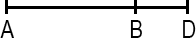A line segment is a portion of a line with a measurable length. The midpoint of a line segment is the point exactly halfway between the endpoints. The midpoint bisects (cuts in half) the line segment.

###### Right Angle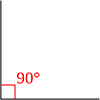A right angle measures 90 degrees and is the intersection of two perpendicular lines. In diagrams, a right angle is indicated by a small box completing a square with the perpendicular lines.

###### Acute & Obtuse Angles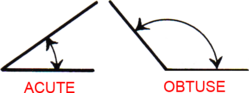An acute angle measures less than 90°. An obtuse angle measures more than 90°.

###### Angles Around Lines & Points

Angles around a line add up to 180°. Angles around a point add up to 360°. When two lines intersect, adjacent angles are supplementary (they add up to 180°) and angles across from either other are vertical (they're equal).

###### Parallel Lines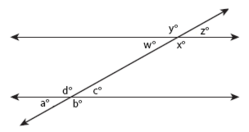Parallel lines are lines that share the same slope (steepness) and therefore never intersect. A transversal occurs when a set of parallel lines are crossed by another line. All of the angles formed by a transversal are called interior angles and angles in the same position on different parallel lines equal each other (a° = w°, b° = x°, c° = z°, d° = y°) and are called corresponding angles. Alternate interior angles are equal (a° = z°, b° = y°, c° = w°, d° = x°) and all acute angles (a° = c° = w° = z°) and all obtuse angles (b° = d° = x° = y°) equal each other. Same-side interior angles are supplementary and add up to 180° (e.g. a° + d° = 180°, d° + c° = 180°).

##### Polygons
###### Triangle Geometry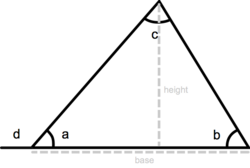A triangle is a three-sided polygon. It has three interior angles that add up to 180° (a + b + c = 180°). An exterior angle of a triangle is equal to the sum of the two interior angles that are opposite (d = b + c). The perimeter of a triangle is equal to the sum of the lengths of its three sides, the height of a triangle is equal to the length from the base to the opposite vertex (angle) and the area equals one-half triangle base x height: a = ½ base x height.

###### Triangle Classification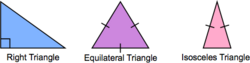An isosceles triangle has two sides of equal length. An equilateral triangle has three sides of equal length. In a right triangle, two sides meet at a right angle.

###### Pythagorean Theorem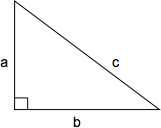The Pythagorean theorem defines the relationship between the side lengths of a right triangle. The length of the hypotenuse squared (c2) is equal to the sum of the two perpendicular sides squared (a2 + b2): c2 = a2 + b2 or, solved for c, $$c = \sqrt{a + b}$$A quadrilateral is a shape with four sides. The perimeter of a quadrilateral is the sum of the lengths of its four sides (a + b + c + d).

###### Rectangle & Square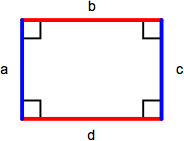A rectangle is a parallelogram containing four right angles. Opposite sides (a = c, b = d) are equal and the perimeter is the sum of the lengths of all sides (a + b + c + d) or, comonly, 2 x length x width. The area of a rectangle is length x width. A square is a rectangle with four equal length sides. The perimeter of a square is 4 x length of one side (4s) and the area is the length of one side squared (s2).

###### Parallelogram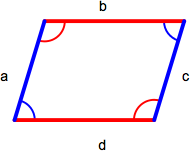A parallelogram is a quadrilateral with two sets of parallel sides. Opposite sides (a = c, b = d) and angles (red = red, blue = blue) are equal. The area of a parallelogram is base x height and the perimeter is the sum of the lengths of all sides (a + b + c + d).

###### Rhombus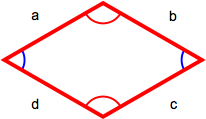A rhombus has four equal-length sides with opposite sides parallel to each other. The perimiter is the sum of the lengths of all sides (a + b + c + d) or, because all sides are the same length, 4 x length of one side (4s).

###### Trapezoid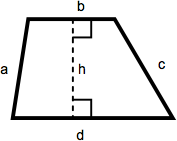A trapezoid is a quadrilateral with one set of parallel sides. The area of a trapezoid is one-half the sum of the lengths of the parallel sides multiplied by the height. In this diagram, that becomes ½(b + d)(h).

##### Circles
###### Dimensions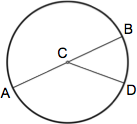A circle is a figure in which each point around its perimeter is an equal distance from the center. The radius of a circle is the distance between the center and any point along its perimeter (AC, CB, CD). A chord is a line segment that connects any two points along its perimeter (AB, AD, BD). The diameter of a circle is the length of a chord that passes through the center of the circle (AB) and equals twice the circle's radius (2r).

###### Calculations

The circumference of a circle is the distance around its perimeter and equals π (approx. 3.14159) x diameter: c = π d. The area of a circle is π x (radius)2 : a = π r2.

##### Solids
###### Cubes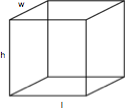A cube is a rectangular solid box with a height (h), length (l), and width (w). The volume is h x l x w and the surface area is 2lw x 2wh + 2lh.

###### Cylinders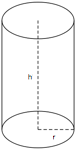A cylinder is a solid figure with straight parallel sides and a circular or oval cross section with a radius (r) and a height (h). The volume of a cylinder is π r2h and the surface area is 2(π r2) + 2π rh.

##### Coordinate Geometry
###### Coordinate Grid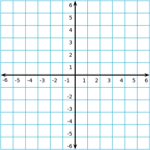The coordinate grid is composed of a horizontal x-axis and a vertical y-axis. The center of the grid, where the x-axis and y-axis meet, is called the origin.

###### Slope-Intercept Equation

A line on the coordinate grid can be defined by a slope-intercept equation: y = mx + b. For a given value of x, the value of y can be determined given the slope (m) and y-intercept (b) of the line. The slope of a line is change in y over change in x, $${\Delta y \over \Delta x}$$, and the y-intercept is the y-coordinate where the line crosses the vertical y-axis.